# Reflect in a Given Line

In this worksheet, students will practise reflecting shapes.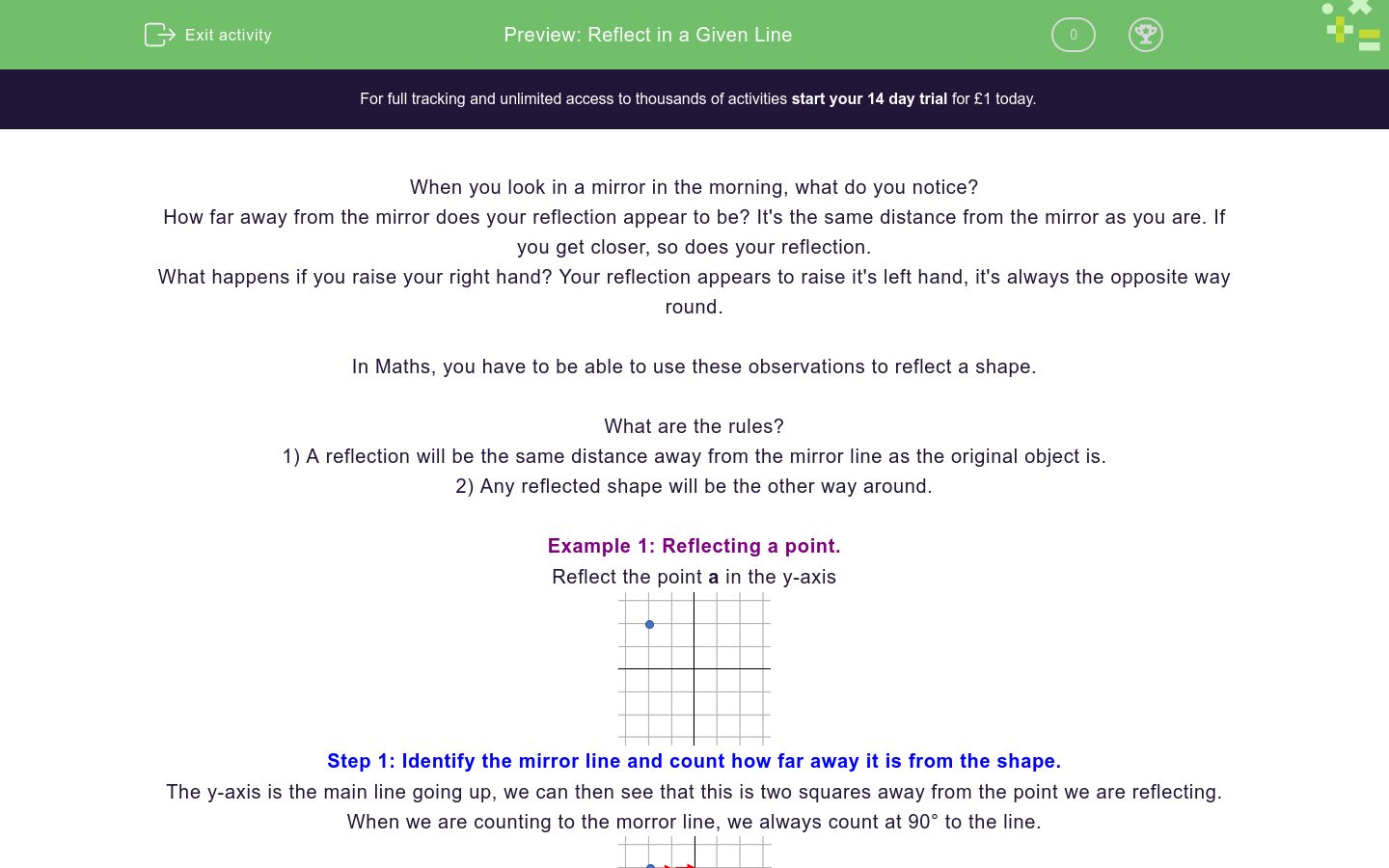Key stage:  KS 4

GCSE Subjects:   Maths

GCSE Boards:   AQA, Eduqas, Pearson Edexcel, OCR

Curriculum topic:   Geometry and Measures, Congruence and Similarity

Curriculum subtopic:   Properties and Constructions, Plane Isometric Transformations

Difficulty level:### QUESTION 1 of 10

When you look in a mirror in the morning, what do you notice?

How far away from the mirror does your reflection appear to be? It's the same distance from the mirror as you are. If you get closer, so does your reflection.

What happens if you raise your right hand? Your reflection appears to raise it's left hand, it's always the opposite way round.

In Maths, you have to be able to use these observations to reflect a shape.

What are the rules?

1) A reflection will be the same distance away from the mirror line as the original object is.

2) Any reflected shape will be the other way around.

Example 1: Reflecting a point.

Reflect the point a in the y-axis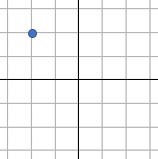Step 1: Identify the mirror line and count how far away it is from the shape.

The y-axis is the main line going up, we can then see that this is two squares away from the point we are reflecting.

When we are counting to the morror line, we always count at 90° to the line.Step 2: Count the same distance from the other side.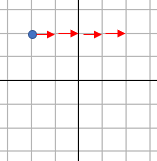Step 3: Draw and label the point.

When we are drawing reflections, we need to be able to tell between the original and the reflected shape.

To do this we use dashes.

If the original is a, the reflection is a'.Example 2: Reflect shape a in the line y = 1.Step 1: Identify the mirror line.

A lot of the time, you will be told to reflect in one of the axes. In this question you have to reflect in the line y = 1.

Remember that the line y = 1 will be a horizontal line that goes through the y - axis at 1.Step 2: Reflect each of the corner points (the vertices) in the new mirror line.

We just do this the same way that we did before, there's just more of them.Step 3: Join up the points and label the shapes.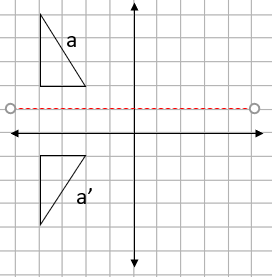When we reflect a shape it will be the...

When we reflect a shape it will be the...

If I reflect the point (2,3) in the x-axis, what will be the coordinates of the reflected point?

(HINT: Try drawing it out on a grid if you find that easier)

Give your answer in the form (a,b), don't put spaces anywhere in the coordinate or the system will mark it as incorrect,

Match the coordinates that are reflections of each other in the x -axis.## Column B

b
i
h
a
e
c
d
g
a
f

Jim completes a reflection question in a Maths lesson.

He has to reflect a shape in the y - axis.

Did her get the answer correct?Yes

No

This triangle needs to be reflected in the y - axis.

Which of these will be valid co-ordinates for the reflection?(1,1)

(-3,-1)

(1,3)

(2,4)

(-1,-1)

(-2,-4)

Match the line with the correct equation.## Column B

a
y = 3
b
y = 1
c
x = -3
d
x = 1

This rectangle is reflected in the line x = 1.What are the coordinates of...

## Column B

a
y = 3
b
y = 1
c
x = -3
d
x = 1

A shape is reflected in a diagonal mirror line.

Which of the two options is the correct reflection?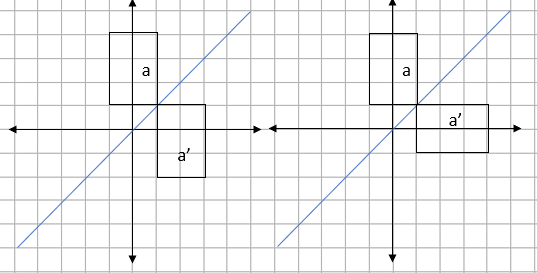Left one

Right one

This rectangle is reflected in the diagonal mirror line that is shown.What are the coordinated of a'?

Left one

Right one

• Question 1

When we reflect a shape it will be the...

EDDIE SAYS
Think back to the example about looking in the mirror, as you get closer, so does the reflection.
• Question 2

When we reflect a shape it will be the...

EDDIE SAYS
Think back to the example about looking in the mirror, if you raise your left hand, the reflection will appear to raise it's right hand.
• Question 3

If I reflect the point (2,3) in the x-axis, what will be the coordinates of the reflected point?

(HINT: Try drawing it out on a grid if you find that easier)

Give your answer in the form (a,b), don't put spaces anywhere in the coordinate or the system will mark it as incorrect,

(2,-3)
EDDIE SAYS
The x-axis is the horizontal main line. If we reflect in this, the x coordinate (the first one) will stay the same and the y-coordinate will change from a positive to a negative.
• Question 4

Match the coordinates that are reflections of each other in the x -axis.## Column B

b
f
h
c
e
i
d
g
a
a
EDDIE SAYS
A nice easy one if you know the trick. If they are reflected in the x axis they are the same distance above and below the line. The one that catches people out is a. If the point is on the line, it does reflect, but to the same point. This is called an invariant point.
• Question 5

Jim completes a reflection question in a Maths lesson.

He has to reflect a shape in the y - axis.

Did her get the answer correct?No
EDDIE SAYS
This comes down to the rules of reflection. 1) Is it the same distance from the mirror line? 2) Is it the opposite way around? Which rule makes this an incorrect reflection.
• Question 6

This triangle needs to be reflected in the y - axis.

Which of these will be valid co-ordinates for the reflection?(1,1)
(1,3)
(2,4)
EDDIE SAYS
This is actually really easy. If you did the reflection, the image should be in the top right corner. This means the coordinates have to both be positive numbers as the image is in the first quadrant.
• Question 7

Match the line with the correct equation.## Column B

a
x = 1
b
y = 3
c
y = 1
d
x = -3
EDDIE SAYS
When we have horizontal and vertical lines, we just need to look where they go through the axis. If a horizontal line goes through the y axis at 2, we call that line y = 2
• Question 8

This rectangle is reflected in the line x = 1.What are the coordinates of...

EDDIE SAYS
The first step here is to find the mirror line. For the mirror line x = 1, we need a vertical line going through the x - axis at 1. We can then just reflect the point a (-3,4) in this line.
• Question 9

A shape is reflected in a diagonal mirror line.

Which of the two options is the correct reflection?Right one
EDDIE SAYS
If you turned the image so the mirror line was in the middle (try turning your head for this), which of the two reflections shows the shape being the opposite way around?
• Question 10

This rectangle is reflected in the diagonal mirror line that is shown.What are the coordinated of a'?

EDDIE SAYS
The mistake people make here is counting in the wrong direction. If your mirror line is diagonal, you need to count diagonally. Counting diagonally to the mirror line is 2.5 squares. If you then count the same distance on the other side, you get to...
---- OR ----

Sign up for a £1 trial so you can track and measure your child's progress on this activity.

### What is EdPlace?

We're your National Curriculum aligned online education content provider helping each child succeed in English, maths and science from year 1 to GCSE. With an EdPlace account you’ll be able to track and measure progress, helping each child achieve their best. We build confidence and attainment by personalising each child’s learning at a level that suits them.

Get started